﻿ Differences Problem Solving Ability Use Model Blended Learning Based Problem on Relation and FunctionsPublications are Open
Access in this journal
Article Versions
Export Article
• Normal Style
• MLA Style
• APA Style
• Chicago Style
Research Article
Open Access Peer-reviewed

### Differences Problem Solving Ability Use Model Blended Learning Based Problem on Relation and Functions

Khairul Ramadhani Daulay , Mulyono, Mariani, Mukhtar, Muliawan Firdaus
American Journal of Educational Research. 2019, 7(11), 742-748. DOI: 10.12691/education-7-11-1
Received September 15, 2019; Revised October 24, 2019; Accepted November 01, 2019

### Abstract

This research aims to identify differences in students problem-solving abilities after applying blended learning based problems and to see the interaction between learning models and early mathematical ability on the student for problem-solving ability. This research was a quasi-experimental study with a sample of 61 students with class VIII-A totaling 30 students as an experimental class and class VIII-B totaling 31 students as a control class. The data to be collected in this study is the data of students' problem solving abilities. The instrument used to collect the data was a problem-solving ability test. The data collected is then analyzed using the two paths ANOVA in the SPSS program. Based on the results of data analysis, it can be concluded that there is a significant difference between problem-based mixed learning models and conventional learning on students' problem-solving abilities.

### 1. Introduction

Education is important and fundamental for every human being. Education is the main capital for the progress of a country. The world of education today has changed a lot in terms of curriculum content to the point where teachers are required to use media in the learning process. In response to these changes, the teacher is not just teaching (transfer of knowledge) but must be a manager in terms of learning. In realizing the goals of national education, the government through schools provides students with various subjects that must be mastered. One of the subjects that has an important role in aspects of life to realize the goals of education is mathematics.

Cocroft 1 said that mathematics needs to be taught to students because: (1) It is always used in all life, (2) All fields of study require appropriate mathematical skills, (3) Is a means of communication that is strong, brief and clear, (4) It can be used to present information in various ways, (5) Increase the ability to think logically, accuracy and awareness of the room, (6) And provide satisfaction with efforts to solve challenging problems.

But the reality is the mathematics learning that is carried out is not as expected. The role of mathematics becomes very calculated because it serves as a servant for other disciplines and can train higher-order thinking skills 2. Based on the 2015 Program for International Student Assessment (PISA) survey, Indonesia ranked 63 out of 72 participating countries with an average score of 386 for mathematics with an average international score of 490 3.

The factors that cause the low performance of Indonesian students in PISA are the weak ability to solve non-routine or high-level problems. This is because during learning students are not given the opportunity to develop their ability to solve problems. Based on its development, the problems faced in learning mathematics increasingly complex and lead to the goals of creative 21st century education, so according to 4, that what is really needed in learning is the ability to understand in mathematics and problem solving in mathematics and communication mathematics, in order to be able to solve mathematical problems.

So the Wertheimer 5 said that the formation of problem-solving abilities in learning can occur because of the discovery of various ways of solving a problem. In mathematics, a problem requires a systematic procedure to solve it. How to solve problems obtained by students is the result of students' knowledge and experience related to problems that students want to find a solution. According to 6 said that problem solving is a process to overcome difficulties encountered to achieve a desired goal.

Factors causing the low ability of students 'mathematical problem solving, influenced by the learning used by teachers so far have not been able to arouse students' enthusiasm for learning, and lack in motivating students to solve problems in the form of problems. The low ability of students to solve problems due to the learning process carried out by teachers in teaching only explains concepts provides examples of questions, questions and answers (if any), followed by asking students to work on similar problems. Based on this students assume that the teacher is the only source of knowledge that causes students to have a dependency on others, especially to the teacher.

At present, the world is entering the era of the industrial revolution of 4.0. or the fourth world industrial revolution where technology has become the basis of human life. Everything becomes infinite and unlimited due to the development of the internet and digital technology. It is hoped that the use of information and communication technology in learning mindsets can shift from being teacher-centered to student-centered.

In the 2013 curriculum itself, the use of technology in learning became something that was highly recommended. The learning process in the 2013 curriculum requires students to actively participate and provide sufficient space for students' creativity, interests and talents. The same thing also stated by 7 that technology becomes something important in learning mathematics, because technology is very influential in improving the process of learning mathematics. With the use of technology, students can learn abstract mathematical objects, as well as students, can make generalizations to conditions in mathematics. In accordance with the times, the learning process has been developed by optimizing learning such as e-learning. E-learning learning is a learning technology that plays an important role in students accessing learning distance.

Based on the above problems, we need a learning model that is able to improve problem-solving abilities One of the learning models that are creative, innovative, and effective in improving problem-solving abilities and student learning independence is Blended learning. Blended learning is part of an effort to use technological advances in improving the quality of learning. Rapid technological advances have influenced the changing world of education and learning.

According to 8 said that Blended Learning is a combination of traditional learning and an electronic learning environment. Blended Learning combines aspects of web/internet-based learning, video streaming, audio communication synchronous and asynchronous with traditional learning or face to face. Blended Learning is basically a combination of learning excellence done face-to-face (face to face learning) and virtually (e-learning).

This statement is supported by 9, Blended Learning is a process of uniting various learning methods that can be achieved by combining virtual and physical resources. By combining virtual and physical resources, learning is expected to be more effective and efficient. Besides this learning offers some convenience because learning by computer (Online) does not completely eliminate face-to-face learning.

So that the implementation of the Blended Learning model is more meaningful than learning that is able to facilitate students towards it is needed. Problem-based learning is felt to be able to direct students to get meaningful learning processes. Problem-based learning is an approach to learning by making confrontations with students with practical problems, in the form of ill-structured, or open-ended through stimulus in learning. By doing these ill-structured solutions, it is hoped that students will be able to form students' mindsets.

According to Tan 10, problem-based learning models are learning innovations, because, in such learning, students' thinking abilities are truly optimized through systematic group or teamwork processes, so students can empower, hone, test and develop their thinking abilities in a way continuous. The concept of finding answers to problems that exist found themselves by students in the group. In the problem-based learning process, each student is required to be responsible for his learning.

This statement is supported by 11 suggests several advantages of problem-based learning, including: problem-based learning is a pretty good technique in understanding the content of the lesson, can challenge the ability of students and provide satisfaction to find new knowledge for students, can increase student activity in learning activities, can help students to transfer knowledge to understand problems in real life, can help students develop new knowledge and be responsible for the learning they do. Problem-based learning can encourage students to make their own evaluations of both the results and the learning process, seen as more exciting and liked by students, can develop students' ability to think critically and develop their ability to solve with new knowledge and problem-based learning can provide opportunities for students to apply knowledge that is already possessed in the real world.

Based on the description above, this study is to see the extent of the impact of problem-based Blended Learning on the problem-solving abilities of VIIII Middle School students. The results of this study are also expected to provide information for teachers, schools and other researchers to further develop students' problem-solving abilities.

### 2. Research Methodology

This research was conducted at Medan Imelda Private Middle School in class VIII in the first semester of the 2018/2019 academic year. This research is included in quasi-experimental research (quasi-experiment). In this study using a test instrument. The test instrument in the form of a description test is used to determine the initial mathematical abilities and problem-solving abilities of students. The design used in this study includes three stages, namely: the validation stage of learning devices and research instruments, the testing phase of learning devices and research instruments, and the stage of conducting experiments. Device validation is focused on content, format, language, and illustrations as well as conformity with problem-based blended learning models. After validation by experts and revisions, the devices and instruments are ready to be trialed. Then the instrument test phase is held to students who are outside the object of research, namely students who have studied the material used. Then the validity, reliability, distinguishing power, and difficulty level of the analysis are carried out.

The validity concerning the accuracy of the measuring instrument against the mastery of the concept, so it is correct to measure what should be measured. To calculate the validity of the item description used the product-moment correlation coefficient formula as follows: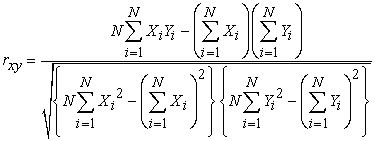Description: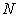: Number of student taking the test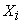Scores Earned on each item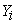Total scored of each item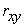Coefficient of validity test.

Next, to determine the validity of a test item, it is used to test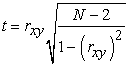with the criteria to be met is if thitung> ttabel with ttabel =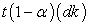for dk = N-2 and α (significant level) is selected 5% The Instrument is valid.

For the suggests that the reliability coefficient of test form description (Essay test) can be used Alpha formula, calculation of the test reliability, 12 as follows: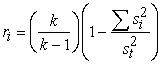Description: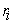: Overall test reliability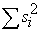: Number of score variances of each problem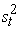: Total Variance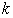: Number of items in the test.

Difficulty Index (Difficulty Index) is the number that indicates the difficulty and ease of a problem 12. Whether or not the item items on the instrument can be known from the degree of difficulty for each item indicates whether the item is classified as difficult, moderate or easy. Determination of the difficulty index is determined by the formula according to the following: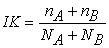Description: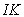: Difficulty index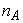: The number of top group students who answered the questions correctly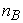: Many lower class students answer correctly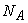: The number of top group students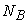: The number of students in the lower classes

A question is said to have a good differentiation if smart students can answer the questions well. The division of upper and lower groups is done by taking 27% for each group. Meanwhile, to calculate the distinguishing power using the following formula: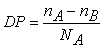Description: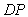: Distinguishing items: The number of top group students who answered the questions correctly: Many lower class students answer correctly: The number of top group students

In connection with the formulation of the research problem, the process of student answers was analyzed by descriptive statistical analysis. Data about student learning outcomes were analyzed with inferential statistics. Descriptive analysis in this study using frequency, average and percentage tables. Data on the results of students' answers to the initial mathematical ability and posttest problem-solving ability were analyzed descriptively to describe the level of problem-solving of students after the implementation of Blended Learning problem-based learning and conventional learning. For this completeness description, the data analyzed are the initial mathematical ability, posttest, and questionnaire data. In this study, the analysis used is quantitative (inferential) analysis. Quantitative analysis is used to analyze data obtained from scores of problem-solving abilities grouped according to problem-based Blended Learning models and conventional learning.

In the initial stage, data processing begins with a descriptive analysis that is calculating the average, standard deviation, maximum value and minimum value of the test data for problem-solving abilities and improvement of these abilities. The second stage of the posttest data was tested using the analysis prerequisite test, the third stage was the hypothesis test.

### 3. Results and Discussion

To answer the problem formulation presented in the introduction, it is necessary to analyze and interpret the data from the results of the study so that it can meet the objectives of this study. The main purpose of this study is to analyze the differences in problem-solving abilities and learning independence of students who are taught using problem-based blended learning models with conventional learning. Also, it is to find out whether there is an interaction between the learning model and the initial ability of mathematics to the ability of problem-solving and student learning independence. The school subject used in this study was Imelda Medan Private Middle School. From the school 2 classes were taken as samples which were used as the experimental class and the control class.

3.1. Research Result

The school subject used in this study was Imelda Medan Private Middle School. From the school 2 classes were taken as samples which were used as the experimental class and the control class.

3.1.1. Description of Beginning Mathematics Ability (KAM) Students

Mathematical initial ability (KAM) is the knowledge students have before learning takes place. The initial ability of mathematics is the foundation as a basic capital for the formation of new concepts in learning. The purpose of this initial mathematical ability is to find out students' high and low mathematical abilities before learning is applied to both the experimental and control classes. Students' initial mathematical abilities are measured through data that has been collected based on diagnostic tests that have been given to both classes. Average summary results and standard KAM deviations as shown in Table 1. the following:

3.1.2. KAM Data Normality Test

One of the requirements in quantitative analysis is the fulfillment of the normal assumption of data distribution to be analyzed. The normality test used in this study uses the Kolmogorov-Smirnov test with a significance level of 5%. The hypotheses tested are:

Ho: Samples come from populations that are normally distributed

Ha: Samples come from populations that are not normally distributed

The summary results of the KAM data normality test calculations are presented in Table 2. as follows:

From Table 2 above shows that the significance values of Kolmogorov Smirnov are 0,185 and 0,200 for the experimental and control classes, respectively. The second significant value of the two classes is greater than the significant level value of 0,05, so the null hypothesis which states the data are normally distributed for both classes can be accepted. In other words, the data for both classes have normally distributed data.

3.1.3. Homogeneity Test for KAM Data

After conducting the normality test, the homogeneity of variance was tested for the experimental class and the control class at a significant level of 5%. The homogeneity test calculation results from both classes are presented in Table 3. The following:

From Table 3 above shows that a significant value of 0,355 is greater than a significant level of 0,05, so the null hypothesis is that there is no difference in variance between groups. This shows that the two data groups of the two classes have homogeneous data variance.

3.1.4. KAM Data Average Similarity Test

The average similarity test was conducted to see the similarity in the average initial ability of mathematics (KAM) of students from the experimental class and the control class obtained from the diagnostic tests that were given. To find the similarity of the average KAM using a t-test at the level obtained t count of 0.968 while the t table of 2.004. Because that is so that the null hypothesis is accepted. This shows that the two data groups of the two classes have the same ability.

Then the students' initial mathematical abilities (high and low) are formed based on the student's KAM. For students who have KAM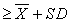classified as high ability, while students who have KAM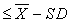are classified as low ability. For the classroom model of problem-based blended learning with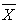= 66.07 and SD = 13.75 so that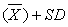=79.82 and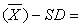52.32. As for the conventional learning class the value= 62.63 and SD = 12.35 so that= 74.97 and50.27. The results of the sample distribution are presented in Table 4. the following:

3.1.5. Description of Post Test Results Troubleshooting Abilities

After applying the learning model to the experimental class, given a post-test the ability to solve problems to determine the extent of students' ability to solve problems after being given learning. Post-test results for both classes are described in Table 5, the following:

Based on Table 5, seen that the minimum value of the post-test problem-solving ability of students in the experimental class was 52, and lower than the control class with a minimum value of 53. For the maximum value of the problem-solving ability of students in the experimental class was 95, and higher than the control class whose maximum score was 89. With thus the average post-test students' problem-solving ability for the experimental class was 79.25, higher than the control class students whose average was 71.67. The standard deviation of the post-test problem-solving ability for the experimental class was 13.67, higher than the control class students with a standard deviation of 10.87.

3.1.6. Data Normality Post Test Problem Solving Ability

The normality test used in this study uses the Kolmogorov-Smirnov test with a significance level of 5%. The output of the normality test post-test data calculation of students' problem-solving abilities is presented in Table 6. below:

Based on Table 6 above shows that the significance value of the post-test data normality test successively is 0.157 and 0.200 for the experimental class and the control class is greater than the significant level of 0.05, so the null hypothesis that states the data are normally distributed for both classes can be accepted. In other words, the data for both classes have normally distributed data.

3.1.7. Data Homogeneity Post Test Problem Solving Ability

Homogeneity testing, in this case, uses the Homogeneity of Variances (Levene Statistics) test at a significance level of 5%. The output of the post-test homogeneity test calculation of students' problem-solving abilities is presented in Table 7 below:

From Table 7, above shows that the significance value of 0.761 is greater than the significance level of 0.05 so that the null hypothesis states there is no difference in variance between groups. This shows that the two data groups of the two classes have homogeneous data variance.

3.1.8. Hypothesis Test

Statistical testing of hypotheses was carried out using two-way ANOVA after the fulfillment of normally distributed data requirements and variance of homogeneous data groups. Based on the results of the post-test problem-solving ability then the following hypothesis test is performed: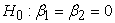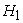there is at least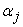one non-zero

Description :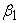: the average problem-solving ability of students taught using the problem-based Blended Learning model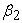: the average problem-solving ability of students taught using conventional learning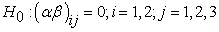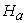there is at least one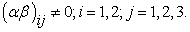Description: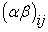is the interaction between learning models and KAM on students' problem-solving abilities.

The results of hypothesis testing with the two-way ANAVA test using the SPSS program can be seen in the following Table 8:

Based on Table 8 above, it can be seen that for the initial mathematical ability (KAM), the Fcount value of 81.149 and a significant value of 0.000 are obtained, because the significant value is smaller than 0.05, reject Ho and accept Ha. So, the KAM category has an effect on problem solving ability. It can also be seen that the learning factor with KAM obtained an F count value of 3.662 and a significant value of 0.033 because the significant value is smaller than 0.05 then reject Ho and accept Ha. Thus, it can be concluded that there is a significant interaction between learning models (problem-based and conventional blended learning) with the initial mathematical ability (KAM) to the problem-solving ability.

This can be interpreted that there is a shared influence given by the learning model and the initial ability of mathematics to the ability of problem-solving.

3.2. Discussion of Research Results

Based on the research findings and the results of data analysis, the relationship will be seen with the stated research objectives. Discussion of the results of the study will be described based on several factors related to this study, namely the factors of the learning model, the ability of problem-solving, and the interaction of the learning model with the initial ability of mathematics to the ability of problem-solving.

The learning model is one of the factors that affect the ability of problem-solving and student learning independence. Each stage in the learning model has a contribution to the ability of problem-solving and student learning independence. Thus, each stage in the problem-based blended learning model is applied in learning in the experimental class, and conventional learning is applied in the control class with the hope of obtaining optimal results.

Problem-based blended learning is learning that emphasizes the real situation or condition of problems and is related to students' daily lives. With the application of problem-based blended learning in learning, students are expected to be able to more easily understand the concepts of what they really want to convey, and can also develop students' existing knowledge to become more complex.

3.2.1. Learning Model Factors

The learning model is one of the factors that affects the ability of problem solving and student learning independence. Each stage in the learning model has a contribution to the ability of problem solving and student learning independence. Thus, each stage in the problem-based blended learning model is applied in learning in the experimental class, and conventional learning is applied in the control class with the hope of obtaining optimal results.

In this study the learning process in each class, the students work together in small groups. This is supported by Vygotsky's learning theory which focuses more on the dialectical relationship between individuals in the formation of such knowledge.

In each class the researcher begins learning through cognitive conflict, this is in line with constructivism learning theory.

3.2.2. Problem-solving Skill

The research conducted at Medan Imelda Private Middle School involved two classes, namely the experimental class in class VIII A and the control class in class VIII B. It is known that prior to conducting research on both classes, the initial step taken by the researcher was to test the instrument for problem-solving ability. After the test run the next stage is given a different treatment in the experimental class and the control class, then both classes are given a post-test to determine the ability of problem-solving after being given treatment. Post-test consists of 5 items that contain aspects and indicators of problem-solving, namely identifying known elements, formulating problems or compiling mathematical models, implementing strategies, explaining or interpreting results, and using mathematical meaningfully.

Based on the results of descriptive data analysis after being given treatment, the problem solving ability of students who study with problem-based blended learning is higher than students who study with conventional learning.

Based on the explanation above, the problem solving ability of students who learn with bledned learning based on problem is higher than students who learn with conventional learning. The results were analyzed with ANOVA Dua Path and it can be concluded that there are differences in the problem solving abilities of students who learn through problem-based blended learning and conventional learning.

The results of this study are in line with 13 entitled The application of blended learning with Edmodo applications based on method learning strategies to improve student achievement concludes the application of blended learning with Edmodo applications based on PDEODE learning strategies can improve student learning achievement in VIIIF class MTs N Magelang.

Other research conducted by 11 entitled the effect of animated video-assisted blended learning models on problem-solving abilities and students' understanding of concepts results in understanding mathematical concepts and problem-solving abilities of students who take blended learning models assisted with video animation more better than understanding mathematical concepts and problem-solving abilities of students who follow traditional models.

3.2.2. The Interaction between Learning Models with Early Mathematical Abilities to Problem Solving Abilities

Interaction is the joint influence of two or more independent variables in influencing the dependent variable. In this case, what is studied is the cooperation between the learning model and the initial mathematical ability to problem-solving skills.

Based on the results of inferential statistical analysis with the two-way ANAVA test on the initial mathematical ability line * the model obtained a sig value of 0.019 <0.05, it can be concluded that there is an interaction between the learning model (problem-based and conventional blended learning) with the initial ability of mathematics to the ability solving student problems.

The interaction between the learning model and the initial mathematical ability of students' problem-solving abilities means that together the learning model and the initial mathematical abilities result in significant differences in the problem-solving abilities of students from the two classes, namely the experimental class and the control class. With the influence of interaction, it cannot be concluded directly that one learning model is better than another. For this reason, further tests are needed on the students' problem-solving ability data.

### 4. Conclusion

Based on the analysis of research data and the discussion of research from the problem-based blended learning model and conventional learning of problem-solving abilities and learning independence of students in class VIII of Imelda Private Junior High School, the researcher obtained several conclusions which were answers to questions raised in the problem statement. The conclusions are as follows:

1) There is a difference in the problem-solving ability of students who are given a model of problem-based blended learning with students who are given conventional learning.

2) There is an interaction between models (problem-based blended learning and conventional learning) seen from the initial ability of mathematics to students' problem-solving abilities. This also means that the interaction between learning models (problem-based blended learning and conventional learning) and initial mathematical abilities (high, medium and low) have had a joint influence on students' problem-solving abilities due to differences in learning used and also because of students' initial mathematical abilities.

### Acknowledgments

The researcher would like to thank Indah Puspita, S.Pd as a teacher of SMP Swasta Imelda Medan, class VIII-A and VIIII-B students of SMP Swasta Imelda Medan who participated in this research. Thank you to my parents who have supported me in writing this article.

### References

  Abdurrahman, M., “Pendidikan Bagi Anak Berkesulitan Belajar”. Jakarta. 2003. In article  Asis, M. Arsyad, N & Alimuddin., “Profil Kemampuan Spasial Dalam Menyelesaikan Masalah Geometri Siswa Yang Memiliki Kecerdasan Logis Matematis Tinggi Ditinjau Dari Perbedaan Gender. Jurnal Daya Matematis. 3 (1). 78-87. 2015. In article View Article  Inayah, S., “Penerapan Pembelajaran Kuantum Untuk Meningkatkan kemampuan Pemecahan Masalah dan Representasi Multipel Matematis Siswa”. Jurnal Pendidikan Matematika. 3 (1). 1-16. 2018 In article View Article  Purba, E. P., “Analysis of the Difficulties of the Mathematical Creative Thinking Process in the Application of Problem Based Learning Model”. AISTEEL. Advances in Social Science, Education and Humanities Research, 104. 265-268. 2017. In article  Wahyuddin., “Pengaruh Metakognisi, Motivasi Belajar, Dan Kreativitas Belajar Terhadap Kemampuan Pemecahan Masalah Siswa Kelas VIII SMP Negeri 2 Sabbangparu Kabupaten Wajo”. Jurnal Daya Matematis, 4 (1) 72-83. 2016. In article View Article  Sumartini, T. S., “Peningkatan Kemampuan Pemecahan Masalah Matematis Siswa melalui Pembelajaran Berbasis Masalah”. Jurnal Musharafa. 8 (3) April. 2016. In article  National Council of Teachers of Mathematics (NCTM)., Principles and Standars for School Mathematics. Resto. 2000. In article  Sjukur.S. B., “Pengaruh Blended Learning Terhadap Motivasi Belajar dan Hasil Belajar Siswa Tingkat SMK”. Jurnal Pendidikan Vokasi. 2 (3). 368-378. November. 2012. In article View Article  Hasbullah., “Blended Learning, Trend Strategi Pembelajaran Matematika Masa Depan”. Jurnal Formatif. 4 (1) ISSN: 2088-351x, 65-70. 2014. In article View Article  Rusman., “Belajar dan Pembelajaran Berbasis Komputer Mengembangkan Profesionalisme Guru Abad 21”. Bandung. 2011. In article  Sudiarta, I., I. W. Sudara., “Pengaruh Model Blended Learning Berbantuan Video Animasi Terhadap Kemampuan Masalah dan Pemahaman Konsep Siswa”. Jurnal Pendidikkan dan Pengajaran. Jillid 49, (2). 48-58. 2016. In article View Article  Arikunto, Suharsimi. ”Dasar-Dasar Evaluasi Pendidikan Edisi 2”. Jakarta. 2013. In article  Ekawati, N. Emmy. “Penerapan Blended Learning dengan Aplikasi Edmodo Berbasis Strategi Pembelajaran PDEODE Untuk Meningkatkan Prestasi Belajar Siswa”. Formatif: Jurnal Ilmiah Pendidikan MIPA. 8 (1). April. 2018, 7-16. 2018. In article View ArticleThis work is licensed under a Creative Commons Attribution 4.0 International License. To view a copy of this license, visit http://creativecommons.org/licenses/by/4.0/